## Number Guessing

By asking a small number of innocent-sounding questions about an unknown number, it is possible to reconstruct the number with absolute certainty (assuming that the questions are answered correctly). Ball and Coxeter (1987) give a number of sets of questions which can be used.

One of the simplest algorithms uses only three questions to determine an unknown number:

1. Tripleand announce if the resultis Even or Odd.

2. If you were told that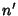is Even, ask the person to reveal the number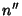which is half of. If you were told thatis Odd, ask the person to reveal the numberwhich is half of.

3. Ask the person to reveal the number of timeswhich 9 divides evenly into.

The original numberis then given byifwas Even, orifwas Odd. For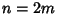even,,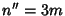,,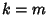, so. Forodd,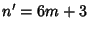,,,, so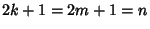.

1. Multiply the numberby 5.

2. Add 6 to the product.

3. Multiply the sum by 4.

4. Add 9 to the product.

5. Multiply the sum by 5 and reveal the result.
The original number is then given by, since the above steps give.

References

Bachet, C. G. Problèmes plaisans et délectables, 2nd ed. 1624.

Ball, W. W. R. and Coxeter, H. S. M. Mathematical Recreations and Essays, 13th ed. New York: Dover, pp. 5-20, 1987.

Kraitchik, M. ``To Guess a Selected Number.'' §3.3 in Mathematical Recreations. New York: W. W. Norton, pp. 58-66, 1942.